# 热防护服的最优厚度设计Optimal Thickness Design of Thermal Protective Clothing

DOI: 10.12677/APP.2019.911057, PDF, HTML, XML, 下载: 314  浏览: 717

Abstract: When working in a high temperature environment, people need to wear special clothing to avoid burns. The special clothing is usually composed of three layers of fabric materials, which are re-ferred to as layers I, II and III, wherein the layer I is in contact with the external environment, and there is a gap between the layer III and the skin, and the gap is recorded as an IV layer. Determin-ing the skin temperature of human body under high temperature operation and the optimal thickness of thermal protective clothing is conducive to designing more reasonable and safe thermal protective clothing. When the human body is in a high temperature environment, wearing thermal protective clothing, respectively, to discuss: 1) When the ambient temperature is 65˚C and the thickness of the IV layer is 5.5 mm, the optimal thickness of the II layer is determined to ensure that the skin of the dummy is 60 minutes after work. The outside temperature does not exceed 47˚C, and the time exceeding 44˚C does not exceed 5 minutes; 2) when the ambient temperature is 80˚C, the optimal thickness of layer II and IV is determined to ensure that the outside temperature of the dummy skin does not exceed 47˚C after working 30 minutes, and more than 44˚C for less than 5 minutes. The finite difference method, least squares method, and bivariate optimization method are used to analyze the data, and then the safety risk coefficient is obtained, and the optimal thickness of each layer of the thermal protective clothing is determined.

1. 引言

1) 根据专用服装材料的参数值，以及假人穿着专用服装(II层厚度为6 mm、IV层厚度为5 mm)在75℃的高温环境中连续作业90分钟，得到假人皮肤外侧每秒温度值，建立数学模型计算各时刻的温度分布。

2) 假人穿着专用服装(IV层的厚度为5.5 mm)在65℃的高温环境中连续作业60分钟，要求假人皮肤外侧温度最高极限为47℃，且超过44℃的时间低于5分钟。在满足以上要求的前提下，确定II层的最优厚度。

3) 假人穿着专用服装在80℃的高温环境中连续作业30分钟，要求假人皮肤外侧温度最高极限为47℃，且超过44℃的时间低于5分钟。在满足以上要求的前提下，确定II层和IV层的最优厚度。

2. 数据获取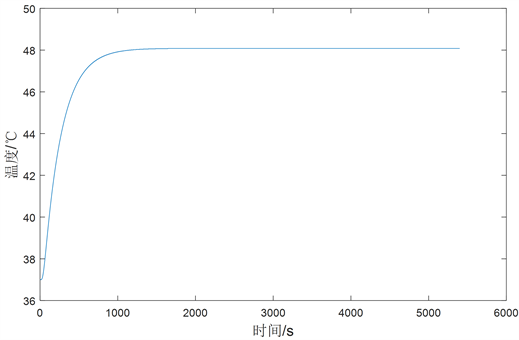Figure 1. Temperature change diagram

· 稳态导热：指物体各点温度不随时间变动的导热过程。

· 非稳态导热：指温度场随时间变化的导热过程。根据其特性可以分为两类非稳态导热：温度场随时间变化趋近于恒定值或温度场随时间变化作周期性变化。

3. 模型建立

3.1. 热传递模型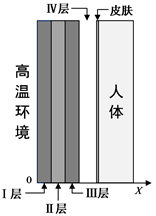Figure 2. Thermal protective clothing-air layer-skin system

· 热传递的方向垂直于皮肤，属于一维非稳态导热情况。

· 外界辐射进入第I层织物后被大量阻隔，因此忽略各织物层之间和空气层的热辐射。

· 空气层厚度值不超过6.4 mm，不考虑热对流的影响。

3.1.1. 传热微分方程的推导

$\frac{\partial e}{\partial t}=-\frac{\partial \varphi }{\partial x}$(1)

$\rho \left(x\right)$ 为质量密度，单位质量的热能为 $c\left(x\right)T\left(x,t\right)$，则单位体积的热能可以表示为

$e\left(x,t\right)=\rho \left(x\right)c\left(x\right)T\left(x,t\right)$(2)

$\rho c\frac{\partial T}{\partial t}=\frac{\partial \varphi }{\partial x}$(3)

$\varphi =-k\frac{\partial T}{\partial x}$(4)

$\rho c\frac{\partial T}{\partial t}=\frac{\partial }{\partial x}\left(k\frac{\partial T}{\partial x}\right)$(5)

3.1.2. 热防护服织物层中的热传递

$\frac{\partial {e}_{L}}{\partial t}=-\frac{\partial {\varphi }_{L}}{\partial x}$

$\beta \left(\sigma {T}^{4}-{\varphi }_{L}\right)=-\frac{\partial {\varphi }_{L}}{\partial x}$

$\frac{\partial {\varphi }_{L}}{\partial x}=\beta {\varphi }_{L}-\beta \sigma {T}^{4}$(6)

$\beta \left(\sigma {T}^{4}+{\varphi }_{R}\right)=\frac{\partial {\varphi }_{R}}{\partial x}$

$\frac{\partial {\varphi }_{R}}{\partial x}=\beta {\varphi }_{R}+\beta \sigma {T}^{4}$(7)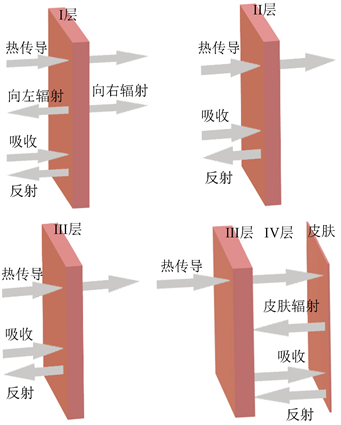Figure 3. Schematic diagram of heat transfer from layer I to layer IV

${\rho }_{\text{I}}{c}_{\text{I}}\frac{\partial T}{\partial t}=\frac{\partial }{\partial x}\left({k}_{\text{I}}\frac{\partial T}{\partial x}\right)+\frac{\partial {\varphi }_{L}}{\partial x}-\frac{\partial {\varphi }_{R}}{\partial x}$$\left(x,t\right)\in \left(0,{L}_{\text{I}}\right)×\left(0,{t}_{\text{exp}}\right)$(8)

${\rho }_{\text{II}}{c}_{\text{II}}\frac{\partial T}{\partial t}=\frac{\partial }{\partial x}\left({k}_{\text{II}}\frac{\partial T}{\partial x}\right)$$\left(x,t\right)\in \left({L}_{\text{I}},{L}_{\text{I}}+{L}_{\text{II}}\right)×\left(0,{t}_{\text{exp}}\right)$(9)

${\rho }_{\text{III}}{c}_{\text{III}}\frac{\partial T}{\partial t}=\frac{\partial }{\partial x}\left({k}_{\text{III}}\frac{\partial T}{\partial x}\right)$$\left(x,t\right)\in \left({L}_{\text{I}}+{L}_{\text{II}},{L}_{\text{I}}+{L}_{\text{II}}+{L}_{\text{III}}\right)×\left(0,{t}_{\text{exp}}\right)$(10)

$T\left(x,0\right)={T}_{1}\left(x\right)$$x\in \left(0,{L}_{3}\right)$(11)

$-{k}_{\text{I}}{\frac{\partial T}{\partial x}|}_{x={L}_{3}}={{q}_{\text{I}}|}_{x=0}$(12)

$-{k}_{\text{III}}{\frac{\partial T}{\partial x}|}_{x={L}_{3}}={\left({q}_{\text{IV}}-{k}_{\text{IV}}\frac{\partial T}{\partial x}\right)|}_{x={L}_{3}}$(13)

$\left(1-{\xi }_{1}\right){\varphi }_{L}\left(0,t\right)+{\xi }_{1}\sigma t{T}^{4}\left(0,t\right)={\varphi }_{R}\left(0,t\right)$

${{T}_{\text{I}}|}_{x={L}_{\text{I}}}={{T}_{\text{II}}|}_{x={L}_{\text{I}}}$

$-{k}_{\text{I}}{\frac{\partial T}{\partial x}|}_{x={L}_{\text{I}}}=-{k}_{\text{II}}{\frac{\partial T}{\partial x}|}_{x={L}_{\text{I}}}$(14)

$\left(1-{\xi }_{2}\right){\varphi }_{L}\left({L}_{\text{I}},t\right)+{\xi }_{2}\sigma t{T}^{4}\left({L}_{\text{I}},t\right)={\varphi }_{R}\left({L}_{\text{I}},t\right)$

${{T}_{\text{II}}|}_{x={L}_{2}}={{T}_{\text{III}}|}_{x={L}_{2}}$

$-{k}_{\text{II}}{\frac{\partial T}{\partial x}|}_{x={L}_{2}}=-{k}_{\text{III}}{\frac{\partial T}{\partial x}|}_{x={L}_{2}}$(15)

3.1.3. 热防护服空气层中的热传递

${\rho }_{\text{IV}}{c}_{\text{IV}}\frac{\partial T}{\partial t}=\frac{\partial }{\partial x}\left({k}_{\text{IV}}\frac{\partial T}{\partial x}\right)-\frac{\partial {q}_{\text{IV}}}{\partial x}$$\left(x,t\right)\in \left({L}_{3},{L}_{4}\right)×\left(0,{t}_{\text{exp}}\right)$(16)

${k}_{\text{IV}}{\frac{\partial T}{\partial x}|}_{x={L}_{3}}={k}_{\text{IV}}{\frac{\partial T}{\partial x}|}_{x={L}_{4}}={h}_{\text{IV}}\left({T|}_{x={L}_{3}}-{T|}_{x={L}_{4}}\right)$(17)

${{q}_{\text{IV}}|}_{x={L}_{3}}={{q}_{\text{IV}}|}_{x={L}_{4}}=\frac{\sigma \left({{T}^{4}|}_{x={L}_{3}}-{{T}^{4}|}_{x={L}_{4}}\right)}{\frac{1}{{\epsilon }_{\text{III}}}+\frac{1}{{\epsilon }_{\text{IV}}}-1}$(18)

$T\left(x,0\right)={T}_{1}\left(x\right)$$x\in \left(0,{L}_{4}\right)$

3.2. 最优厚度模型

I. 决策变量

II. 目标函数

$f\left(t\right)={\int }_{0}^{t}P{\text{e}}^{-\frac{\Delta E}{RT\left(x,t\right)}}\text{d}t$(19)

$J\left({L}_{\text{II}}\right)={\int }_{0}^{t}{|f\left({L}_{\text{II}},t\right)-{f}^{*}|}^{2}\text{d}t$

$J\left({L}_{\text{II}}^{*}\right)=\mathrm{min}J\left({L}_{\text{II}}\right)={\sum }_{i=1}^{n}{\sum }_{j=1}^{m}{\left({f}_{i}^{j}-{f}^{*}\right)}^{2}$

III. 约束条件

$f\left({L}_{\text{II}},t\right)\le 1$

$t\le 5$

$0.6\le {L}_{\text{II}}\le 25$

IV. 最终模型

$\begin{array}{l}\mathrm{min}J\left({L}_{\text{II}}\right)={\sum }_{i=1}^{n}{\sum }_{j=1}^{m}{\left({f}_{i}^{j}-{f}^{*}\right)}^{2}\\ \text{s}.\text{t}.\text{\hspace{0.17em}}\text{\hspace{0.17em}}\text{\hspace{0.17em}}\text{\hspace{0.17em}}f\left({L}_{\text{II}},t\right)\le 1\\ \text{\hspace{0.17em}}\text{\hspace{0.17em}}\text{\hspace{0.17em}}\text{\hspace{0.17em}}\text{\hspace{0.17em}}\text{\hspace{0.17em}}\text{\hspace{0.17em}}\text{ }\text{ }t\le 5\\ \text{\hspace{0.17em}}\text{\hspace{0.17em}}\text{\hspace{0.17em}}\text{\hspace{0.17em}}\text{\hspace{0.17em}}\text{\hspace{0.17em}}\text{\hspace{0.17em}}\text{ }0.6\le {L}_{\text{II}}\le 25\end{array}$(21)

3.3. 双变量优化模型

I. 决策变量

II. 目标函数

$f\left(t\right)={\int }_{0}^{t}P{\text{e}}^{-\frac{\Delta E}{RT\left(x,t\right)}}\text{d}t$

$J\left(L\right)={\int }_{0}^{t}{|f\left(L,t\right)-1|}^{2}\text{d}t$(22)

$J\left(L\right)=\frac{1}{N}{\sum }_{j=1}^{N}{|f\left(L,t\right)-1|}^{2}$

III. 约束条件

$f\left(L,t\right)\le 1$

$t\le 5$

$0.6\le {L}_{\text{II}}\le 25$

$0.6\le {L}_{\text{IV}}\le 6.4$

IV. 最终模型

$\begin{array}{l}\mathrm{min}J\left(L\right)=\frac{1}{N}{\sum }_{j=1}^{N}{|f\left(L,t\right)-{f}^{*}|}^{2}\\ \text{s}.\text{t}.\text{\hspace{0.17em}}\text{\hspace{0.17em}}\text{\hspace{0.17em}}f\left(L,t\right)\le 1\\ \text{\hspace{0.17em}}\text{\hspace{0.17em}}\text{\hspace{0.17em}}\text{\hspace{0.17em}}\text{\hspace{0.17em}}\text{\hspace{0.17em}}\text{ }t\le 5\\ \text{\hspace{0.17em}}\text{\hspace{0.17em}}\text{\hspace{0.17em}}\text{\hspace{0.17em}}\text{\hspace{0.17em}}\text{ }\text{\hspace{0.17em}}0.6\le {L}_{\text{II}}\le 25\\ \text{\hspace{0.17em}}\text{\hspace{0.17em}}\text{\hspace{0.17em}}\text{\hspace{0.17em}}\text{\hspace{0.17em}}\text{ }\text{\hspace{0.17em}}0.6\le {L}_{\text{IV}}\le 6.4\end{array}$(23)

4. 模型的求解

4.1. 问题一的求解

${\rho }_{\text{I}}{c}_{\text{I}}\frac{\partial T}{\partial t}=\frac{\partial }{\partial x}\left({k}_{\text{I}}\frac{\partial T}{\partial x}\right)+\frac{\partial {\varphi }_{L}}{\partial x}-\frac{\partial {\varphi }_{R}}{\partial x}$$\left(x,t\right)\in \left(0,{L}_{\text{I}}\right)×\left(0,{t}_{\text{exp}}\right)$

${\rho }_{\text{II}}{c}_{\text{II}}\frac{\partial T}{\partial t}=\frac{\partial }{\partial x}\left({k}_{\text{II}}\frac{\partial T}{\partial x}\right)$$\left(x,t\right)\in \left({L}_{\text{I}},{L}_{2}\right)×\left(0,{t}_{\text{exp}}\right)$

${\rho }_{\text{III}}{c}_{\text{III}}\frac{\partial T}{\partial t}=\frac{\partial }{\partial x}\left({k}_{\text{III}}\frac{\partial T}{\partial x}\right)$$\left(x,t\right)\in \left({L}_{2},{L}_{3}\right)×\left(0,{t}_{\text{exp}}\right)$

${\rho }_{\text{IV}}{c}_{\text{IV}}\frac{\partial T}{\partial t}=\frac{\partial }{\partial x}\left({k}_{\text{IV}}\frac{\partial T}{\partial x}\right)-\frac{\partial {q}_{\text{IV}}}{\partial x}$$\left(x,t\right)\in \left({L}_{3},{L}_{4}\right)×\left(0,{t}_{\text{exp}}\right)$

${\rho }_{\text{I}}{c}_{\text{I},i}^{j+1}\frac{{T}_{i}^{j+1}-{T}_{i}^{j}}{\tau }=\frac{{k}_{\text{I},i+1}^{j+1}-{k}_{\text{I},i}^{j+1}}{h}\frac{{T}_{i+1}^{j+1}-{T}_{i}^{j+1}}{h}+{k}_{\text{I},i}^{j+1}\frac{{T}_{i-1}^{j+1}-2{T}_{i}^{j+1}+{T}_{i+1}^{j+1}}{{h}^{2}}+{\theta }_{i}^{j+1}$

$-{k}_{\text{I},0}^{j+1}\frac{{T}_{1}^{j+1}-{T}_{0}^{j+1}}{h}={h}_{1}\left({T}_{g}-{T}_{0}^{j+1}\right)$

$-{k}_{\text{I},{m}_{1}}^{j+1}\frac{{T}_{{m}_{1}}^{j+1}-{T}_{{m}_{1}-1}^{j+1}}{h}=-{k}_{\text{II},{m}_{1}}^{j+1}\frac{{T}_{{m}_{1}+1}^{j+1}-{T}_{{m}_{1}}^{j+1}}{h}$Figure 4. Flow chart of the finite difference methodTable 1. Table of material temperature distribution of each layer at part time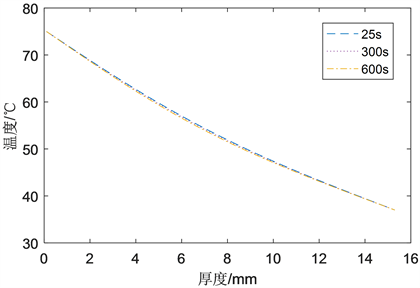Figure 5. Two-dimensional map of temperature distribution at t = 25 s, t = 300 s and t = 600 s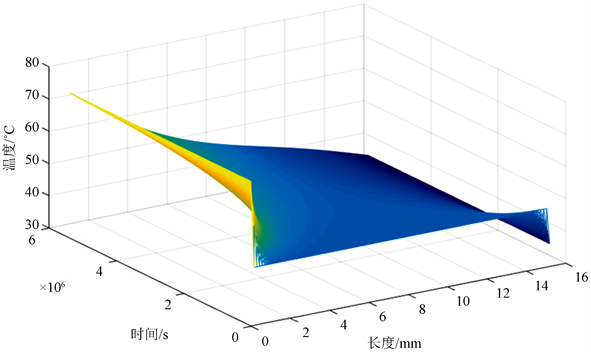Figure 6. 3D map of the temperature distribution of each layer of material

4.2. 问题二的求解

$f=0.0069{L}^{2}-0.1102L+0.4377$.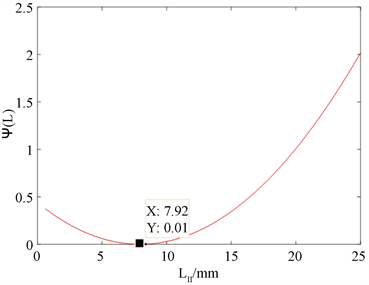Figure 7. Curve fitting of $f-{L}_{\text{II}}$

4.3. 问题三的求解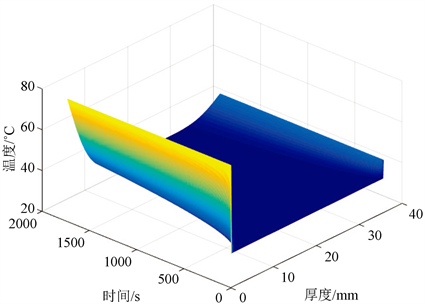Figure 8. Temperature profile at an ambient temperature of 80˚C

${L}_{\text{IV}}={L}_{总}-{L}_{\text{I}}-{L}_{\text{II}}-{L}_{\text{III}}=5.17\text{\hspace{0.17em}}\text{mm}$

5. 误差分析

$f\left({L}_{\text{II}},t\right)<0.53$

$\begin{array}{l}\mathrm{min}J\left({L}_{\text{II}}\right)={\sum }_{i=1}^{n}{\sum }_{j=1}^{m}{\left({f}_{i}^{j}\left({L}_{\text{II}},t\right)-{f}^{*}\right)}^{2}\\ \text{s}.\text{t}.\text{\hspace{0.17em}}\text{\hspace{0.17em}}\text{\hspace{0.17em}}\text{ }\text{ }f\left({L}_{\text{II}},t\right)<0.53\\ \text{\hspace{0.17em}}\text{\hspace{0.17em}}\text{\hspace{0.17em}}\text{\hspace{0.17em}}\text{\hspace{0.17em}}\text{\hspace{0.17em}}\text{ }\text{\hspace{0.17em}}t\le 5\\ \text{\hspace{0.17em}}\text{\hspace{0.17em}}\text{\hspace{0.17em}}\text{\hspace{0.17em}}\text{\hspace{0.17em}}\text{\hspace{0.17em}}\text{\hspace{0.17em}}0.6\le {L}_{\text{II}}\le 25\end{array}$

6. 结论

7. 模型评价与推广

7.1. 模型的优点

1) 对三层织物材料进行了充分分析，建立热传递模型，能够比较准确地计算出任意时间任意坐标点处的温度，从而判断出身着高温作业专用服的工作人员可以在不同温度下安全工作的最大时间；

2) 主要考虑了热辐射和热传导两种热传递方式，比较全面准确地计算了热量的传导和辐射。同时，考虑了高温作业专用服与皮肤之间的空隙，使模型更贴合实际情况；

3) 模型考虑了一维情况的热传递，一定程度上简化了模型和计算，操作更加便捷清晰。

7.2. 模型的缺点

1) 简单地将假人当作圆柱进行建模，没有考虑人体的实际情况来构建仿真人体模型，同时，忽略了人体可以通过出汗等方式散热，可能会对皮肤温度的变化造成影响；

2) 只考虑了热传递，忽略了空气中可能存在水蒸汽，会对热传递产生影响，在实际应用中可能会导致误差。

7.3. 模型的推广

  潘斌. 热防护服装热传递数学建模及参数决定反问题[D]: [硕士学位论文]. 杭州: 浙江理工大学, 2017.  卢琳珍, 徐定华, 徐映红. 应用三层热防护服热传递改进模型的皮肤烧伤度预测[J]. 纺织学报, 2018, 39(1): 111-118+125.  卢琳珍. 多层热防护服装的热传递模型及参数最优决定[D]: [硕士学位论文]. 杭州: 浙江理工大学, 2018.  周远, 徐映红, 徐定华. 结合粒子群算法的一类双层纺织材料厚度设计反问题[J]. 纺织学报, 2013, 34(6): 40-45.  朱方龙, 张渭源. 基于人体皮肤热模型的热防护服评价方法研究[J]. 中国安全科学学报, 2007(11): 134-140+177.  史策. 热传导方程有限差分法的MATLAB实现[J]. 咸阳师范学院学报, 2009, 24(4): 27-29+3.